Home » Getting into Top 10 in Kaggle Facebook Recruiting Competition

# Getting into Top 10 in Kaggle Facebook Recruiting Competition

Facebook recently wrapped up its Recruitment competition on Kaggle. This was by far the richest data I have seen on Kaggle. The amount of information which was available in this competition was simply beyond imagination. Here is what the problem statement looked like :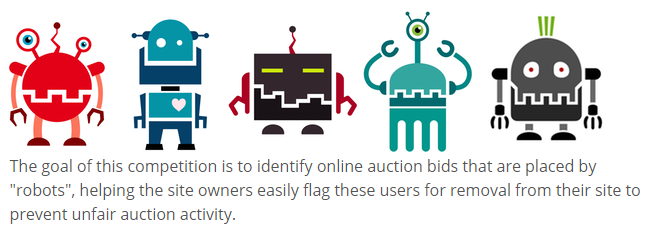Interesting enough? If not, here is what will make you jump on the table : You get the Bid level interaction data of these bidders for a significant time to identify who among the bidders is bot. I performed reasonably well on the public board, however the private board did no justice to my entry on Kaggle. Here was my final standing on the Public board :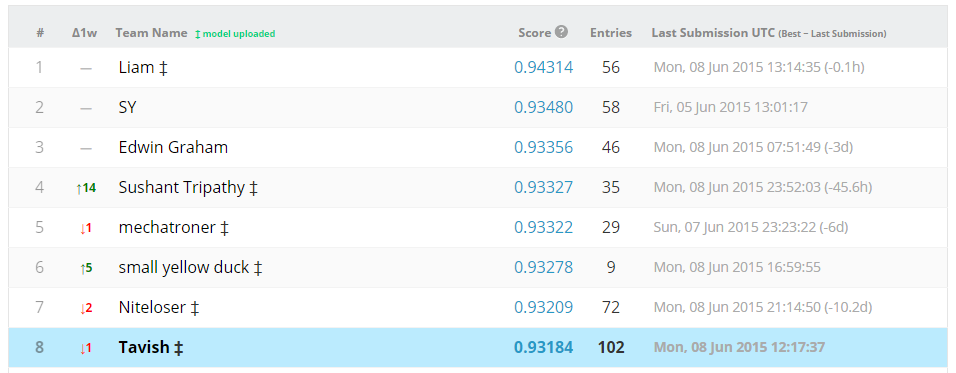Even though I did not win the competition, I thought it will be interesting to share my approach in public.

## Understanding the Data

The data is primarily composed of two tables. One is a simple list of all bidders and the second one contains all the bids made by these bidders. Here are the details of the variables in these two tables :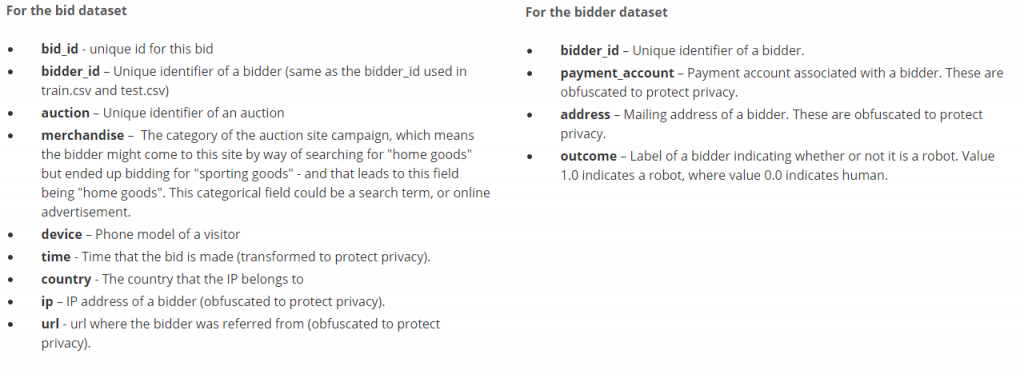By combining the two datasets on Bidder ID, imagine the richness of this data.

## Getting started

To do any kind of prediction and apply machine learning, we need to summarize the data on Bidder ID level. This is because bidder and not bids can be bots. Hence, target variable is defined on bidder level. The simplest way to get do this is simply roll up the bid level information and get the features right away. This was the quick and dirty approach I took at the first go.

### List of initial hypothesis:

Here are the top hypothesis which I had generated before starting with the problem. This approach clearly helped me in identifying bots among the bidders :

1. # of Bids in total : This looked like a long shot but Robots might have significantly higher bids than humans.
2. #Bids / auction : This was an interesting hypothesis. A robot will be more consistent in bidding to win every auction. Hence, robots will have a higher number of bids every auction
3. Response time : Time between two bids will be considerably low for Robots than humans
4. #Distinct IPs/Countries : Robots will probably make bids from multiple IPs and Countries
5. Merchandise : A few merchandise will have more robots bidding.

However, it is purely upto your imagination what variables do you want to create while summarizing it. I created following variables for my model :

```"bids"  : Total number of Bids
"min_resp" : Minimum time between two responses
"mean_resp" : Mean time to make a response
"instant_resp" : Number of instantaneous responses (Responses done on same time)
"url_sum"  : Bids coming out from a particular Domain
"no_auctions" : Distinct auctions the bidder participated in
"no_devices" : Distinct devices used by the bidder
"no_countries" : Number of countries the bidder participated from
"no_ips" : Number of IPs bidder participated with
"merchandise" : Type of Merchandise bidder participated
"Per_instant_resp" : Percent of instantaneous response
"Bids_per_auction" : Bids made in every auction
"Bids_per_device" : Bids made per device
"Bids_per_country" : Bids made per country
"Bids_per_IP" : Bids made per IP```

## Results of initial exploratory analysis

Here are a few insights you will find in the initial analysis

1. Every bidder only bids on a single merchandise. Hence the number of unique bidder-merchandise pair is same as the number of unique bidders.
2. Response time of Robots was found much lesser than Humans.
3. Response rate of the population was quite low but any kind of over sampling is not required because the evaluation criterion is AUC, which simply depends on rank ordering and not the probabilities.
4. I derived a new variable as the bidder which places the bid first and last for every auction. This variable was found to be significant in a few pockets. We can think of this as the the winner of the bid, as I do not see a point of bidding after the winner places the bid.

Here are a few interesting plots which made things simpler on the modeling side :

1. Density plot for mean response time : As you can see from below chart, Robots have some fixed time lag between their bids. However, humans take non-regular time between bids. This looks very intuitive as robots will be programmed to bid and hence show a common pattern.2. Average number of bids : Probability that a bidder is human increases as the number of total bids increase.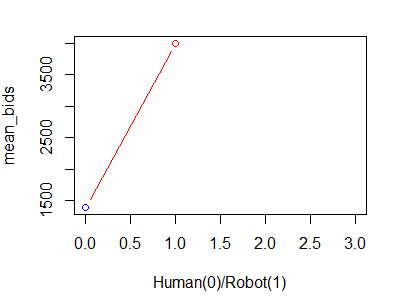## Finding the Significant Variables

Finding variables which are able to distinguish humans from robots is the first thing you will do before building any kind of model. Here is a simple way to do this :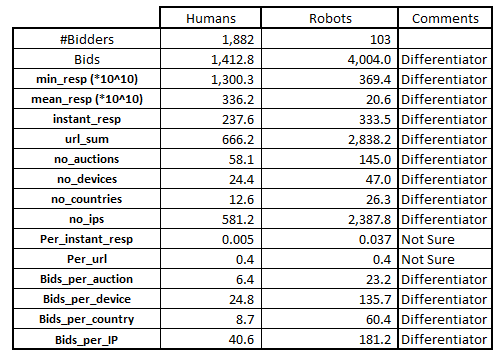Here, what we simply do is find the mean value of each parameter for both humans and Robots and see if they look different. There are many other ways to do the same thing, however this is the simplest of them all the understand. As you can see from the table, most of the variable come out to be significant.

## Developing a simplistic model

Now, once we have a list of significant variables, we simply need to plug them in a machine learning algorithm and check its accuracy with k-folds. I’ve used Gradient Boosting to do this exercise. Here is a simple function which you can use to evaluate your K-Folds accuracy by feeding in any number of variables.

```gbmfold <- function(seed,listoffactors,input,training) {
library(caret)
library(Metrics)
k = 5
set.seed(7)
training\$id <- sample(1:k,nrow(training),replace = TRUE)
list <- 1:k
perf <- vector(mode = "numeric",length=k)
for (i in 1:k) {
trainingset <- subset(training, id %in% list[-i])
testingset <- subset(training, id %in% c(i))
set.seed(seed)
gbmFit1 <- train(as.formula(paste("outcome ~",paste(listoffactors,collapse="+")))
, data = trainingset, method = "gbm", verbose = FALSE)
preds <- predict(gbmFit1, testingset)
perf[i] = auc(preds,testingset\$outcome)
print (perf[i])
}
a <- c(0,0,0)
a <- mean(perf)
a <- min(perf)
a <- max(perf)
if (input == 1) { return(a)
} else {return(gbmFit)}
}```

For using this function simply use following statement

`gbmfold(321,c("bids","mean_resp"),1,train)`

Compare the performance of different models and once you have the final selected variables use it with entire dataset.

For Beginners: How to choose the right Kaggle competition?

## Here is my secret sauce

I soon realized the the CV for different seeds of the same model is varying too much because we train the model on merely 2000 datapoints. So, I took a shot in experimenting over the problem with a non-traditional approach. Here is what I did :

Broke down the bidder IDs with a constant duration of time. For instance if I have time varying from 1 to 100, I divide this time into 10 time points. Now I roll up the bidders using their bidder id and the time point. The assumption being that the observations will be still independent of each other. For instance, I (as a human) might be bidding very aggressively in 2010 but playing safe in 2012. Now I use the same bidder twice with outcome flag as 0 (human).

With this manipulation, I was able to raise the number of observations to around 10,000 and was able to make a CV which gave consistently the same score (which was 0.02% lower than Kaggle leadership board).

Kaggle Trick: Simple framework to crack a Kaggle Problem

### End Notes

My model performed similar on Public and Private scoreboard of Kaggle ( 0.92435 on Public board and 0.92680 on Private board). I think the model looks stable enough and not a case of over fitting. However the winner of the competition had the private score far better than the public score (0.91946 on Public board and 0.94254 on Private board).

If the two samples were similar (Private and Public), is this not a case of unstable model. I reserve my doubts on this issue but will like to encourage people to give their view point on this. Let me know your thoughts on this.

Have you participated in any Kaggle problem?  Did you see any significant benefits by doing the same? Do let us know your thoughts about this guide in the comments section below.

### If you like what you just read & want to continue your analytics learning, subscribe to our emails, follow us on twitter or like our facebook page.

You can also read this article on our Mobile APP•Amit says:

Thanks for this. Is there a way i can attempt this problem now and check the accuracy of the solution?

•Tavish says:

Yes. You can still upload the solution on Kaggle

•Peter says:

Did they send you an invite for FB interview?

•dsai.500 says:

Thanks a lot Tavish.

•sandhya says: